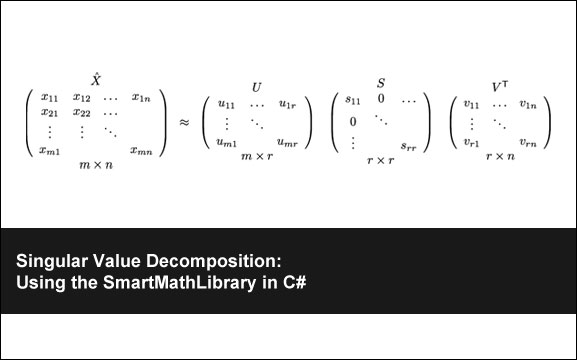## Singular Value DecompositionOctober 26th, 2009  |  Published in Uncategorized  |  3 Comments

Singular Value Decomposition is something I’ve been wanting to wrap my head around for a while now that I am getting really into Machine Learning. Unfortunately, a lot of the material out there is often hard to understand and believe it or not there are few libraries that are available in .NET.

So what is singular value decomposition (SVD)? Probabably, the best description I’ve run across is:

Singular Value Decomposition is a way of factoring matrices into a series of linear approximations that expose the underlying structure of the matrix. SVD is extraordinarily useful and has many applications such as data analysis, signal processing, pattern recognition, image compression, weather prediction, and Latent Semantic Analysis. [iMetaSearch]

SVD formula is:

M=Uâˆ‘V*

M is simply a m-by-n matrix, U form a set of orthonormal “output” basis vector directions for M, Î£ are the singular values, which can be thought of as scalar “gain controls” by which each corresponding input is multiplied to give a corresponding output and V* form a set of orthonormal “input” or “analysing” basis vector directions for M. The best walk through I’ve come across is over at iMetaSearch here.

A lack of good .NET Libraries.

I tried out four different libraries: SmartMathLibrary, LatoolNet, ALGLIB and DotNetMatrix. Out of these four I could only get two of them completely working and I ultimately came to the conclusion that SmartMathLibrary was the best for doing SVD.

The Code

Here is the code to replicate this tutorial over at MIT.

```using System;
using SmartMathLibrary;

namespace MatrixTest2
{
internal class Program
{
private static void Main(string[] args)
{
SVDTest();
}

private static void SVDTest()
{
var holeDifficulty = new double[,]
{
{2, 1, 0, 0},
{4, 3, 0, 0}
};

var a = new Matrix(holeDifficulty);

// Singular Value Decomposition
var SVD = new SingularValueDecomposition(a);
SVD.ExecuteDecomposition();

// Get the general vector
GeneralVector s = SVD.S;

// Display results
Console.WriteLine(a.Transpose().ToString());
Console.WriteLine();
Console.WriteLine(s.ToString());
Console.WriteLine();
Console.WriteLine(SVD.U.ToString());
Console.WriteLine();
Console.WriteLine(SVD.V.ToString());
}
}
}
```

iMetaSearch

IR Math with Java : TF, IDF and LSI

SVD Tutorial

### Related Posts

Starting a Ph.D. in Computer Science
Monte Carlo Simulations in C#
Cryptanalysis Using n-Gram Probabilities
Apriori Algorithm
K-Means Document Clustering